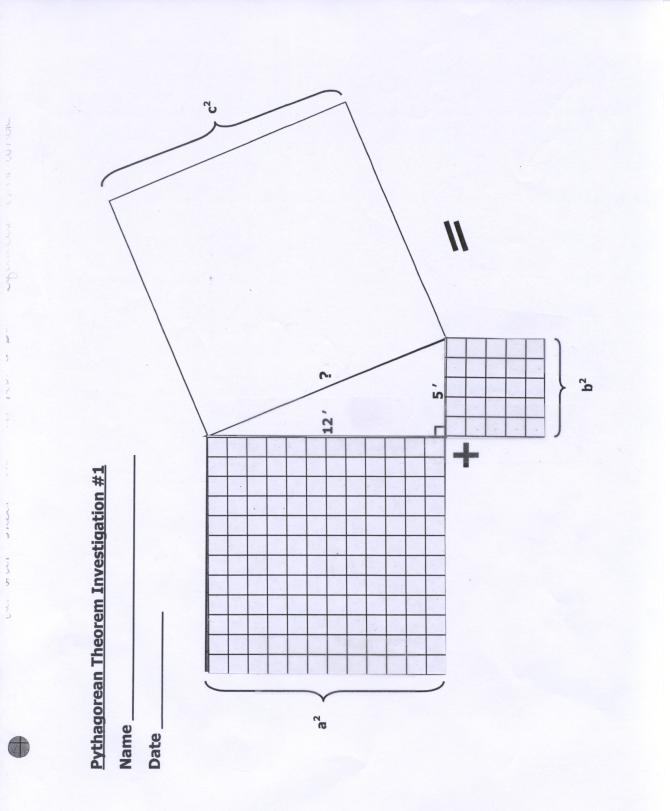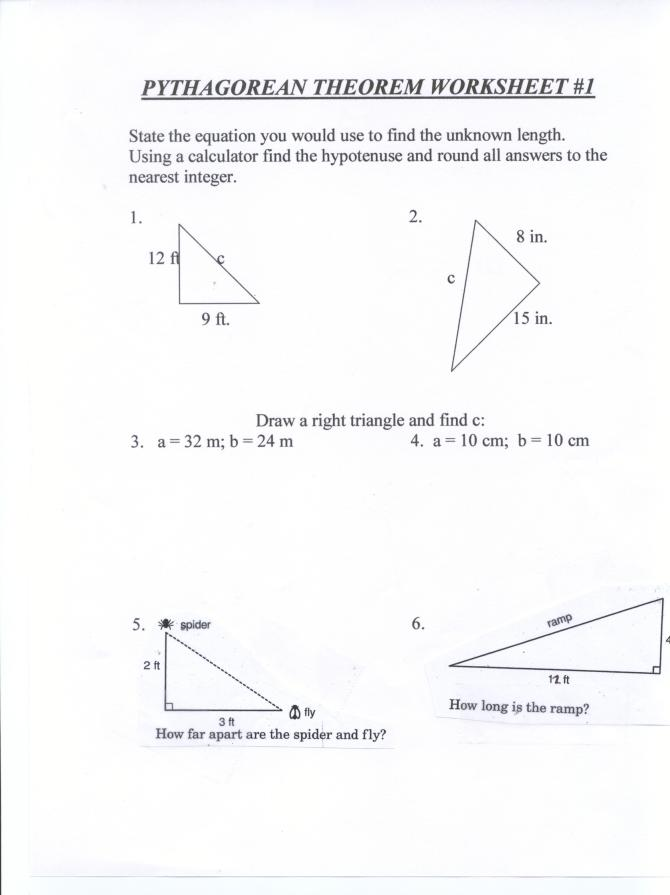#Hello, Guest!

## Pythagorean Theorem by ECSDM

### Subject

Math, Science & Technology

### Objectives

Students will be able to:

• Identify the right angle, hypotenuse and legs of a right triangle.
• Explore the relationship between the lengths of the three sides of a right triangle using the area formulas of squares in a hands-on activity using manipulatives.
• Use the Pythagorean Theorem to determine the unknown length of a hypotenuse of a right triangle by completing the homework assignment.

### Duration

2 (40-minute) class periods

### Description

In this lesson students will discover the Pythagorean Theorem. Students will also use the Pythagorean Theorem to solve real life problems.

### Materials

• SMART Board
• Internet-connected computer
• Scissors

•• Questions of the Day
• Right Triangle Problems #1

•• Questions of the Day.pdf
• ### Procedure

1. As students enter the classroom, have them begin the "Question of the Day" activity.
2. Distribute the worksheet titled "Pythagorean Theorem Investigation #1".
• Locate the right triangle and state the measurement of the legs.
• Find the area of each leg.
• Discover the length of the hypotenuse by cutting out the areas of the two legs.
3. Observe this discovery lesson on the PBS-Pythagorean Puzzle website (Shockwave version).
4. Provide the students with examples where the student will find the hypotenuse given the measurement of right triangles, using the "Right Triangle Problems #1" worksheet.
5. Use the website to show real-life situations involving a baseball field and ladders leaning on a house
6. Assign the "Pythagorean Theorem Worksheet #1" as homework.

### SMART Board

This instructional content was intended for use with a SMART Board. The .xbk file above can only be opened with SMART Notebook software. To download this free software from the SMART Technologies website, please click here.